﻿ Application and principle of temperature sensor (thermistor, thermocouple)_

China sensor manufacturers

# Application and principle of temperature sensor (thermistor, thermocouple)

Temperature measurement applications are very extensive, not only do production processes require temperature control, but some electronic products also need to measure their own temperature. For example, if the computer wants to monitor the temperature of the CPU, the motor controller should know the temperature of the power driver IC, etc. Several commonly used temperature sensor products are described below.
Temperature is a parameter that is often tested in practical applications. From steel manufacturing to semiconductor production, many processes rely on temperature, and temperature sensor products are the bridge between the application system and the real world. This article provides a brief overview of the different temperature sensor products and describes the interface to the circuit system.

Thermistor
There are many types of sensor products for measuring temperature, and thermistors are one of them. Many thermistors have a negative temperature coefficient (NTC), which means that their resistance increases as the temperature drops. In all passive temperature sensor products, the sensitivity of the thermistor (ie, the change in resistance for each degree of temperature change) is highest, but the resistance/temperature curve of the thermistor is non-linear.
Table 1 is a typical NTC thermistor performance parameter.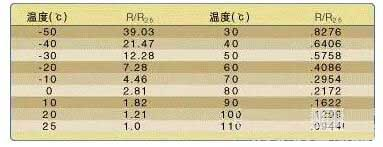These data are measured on a Vishay-Dale thermistor, but it also represents the overall condition of the NTC thermistor. The resistance value is given as a ratio (R/R25), which represents the ratio of the resistance at the current temperature to the resistance at 25 °C. Usually the same series of thermistors have similar characteristics and the same resistance/temperature curve. Take the thermistor series in Table 1 as an example. The resistance at 10 °C is 10KΩ. The resistance is 28.1KΩ at 0 °C. The resistance at 4.0 ° C is 4.086 KΩ; Similarly, a 5 kΩ thermistor at 25 °C has a resistance of 14.050 KΩ at 0 °C.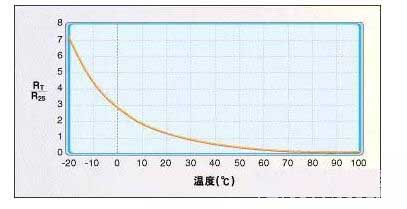Fig. 1 shows the temperature curve of thermistor. It can be seen that the resistance/temperature curve is non-linear. Although the thermistor data here is in increments of 10 ° C, some thermistors can be in increments of 5 ° C or even 1 ° C.  If you want to know the resistance value at a certain temperature between two points, you can use this curve to estimate, or you can directly calculate the resistance value. The calculation formula is as follows: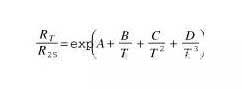Here T refers to the absolute temperature of Kelvin, and A, B, C, and D are constants, which vary according to the characteristics of the thermistor. These parameters are provided by the manufacturer of the thermistor.
Thermistors generally have an error range that is used to specify the consistency between the samples. The error value is usually between 1% and 10% depending on the material used. Some thermistors are designed to be interchangeable for applications where field adjustment is not possible. For example, an instrument, user or field engineer can only replace the thermistor and cannot be calibrated. This thermistor is much higher than the ordinary one and is much more expensive.
Figure 2 is a typical circuit for measuring temperature using a thermistor. Resistor R1 pulls the thermistor's voltage up to the reference voltage, which is generally consistent with the ADC's reference voltage, so if the ADC's reference voltage is 5V, Vref will also be 5V. The thermistor and the resistor are connected in series to generate a partial voltage, and the resistance change causes the voltage at the node to also change. The accuracy of the circuit depends on the error of the thermistor and the resistor and the accuracy of the reference voltage.Self-heating problem
Since the thermistor is a resistor, a certain amount of heat is generated when current flows through it. Therefore, the circuit designer should ensure that the pull-up resistor is large enough to prevent the thermistor from self-heating, otherwise the system measures the heat generated by the thermistor rather than the ambient temperature.

The effect of the energy consumed by the thermistor on temperature is expressed in terms of the dissipation constant, which is the number of milliwatts required to increase the thermistor temperature by 1 °C above ambient temperature. The dissipation constant is different depending on the thermistor package, pin specifications, encapsulation material, and other factors.

The amount of self-heating and current limiting resistor allowed by the system is determined by the measurement accuracy. The measurement system with a measurement accuracy of ±5 °C is larger than the self-heating of the thermistor that the measurement system can withstand with a precision of ±1 °C.

It should be noted that the resistance of the pull-up resistance must be calculated to limit the self-heating power consumption in the whole measuring temperature range. When the resistance value is given, the dissipative power varies at different temperatures due to the variation of the resistance value of thermistor.
It is sometimes necessary to calibrate the input of the thermistor to get the proper temperature resolution. Figure 3 is a circuit that extends the temperature range of 10 to 40 ° C to the entire 0 to 5 V input range of the ADC.

The operational amplifier output formula is as follows:
Once the input of the thermistor is calibrated, the actual resistance to temperature can be graphically represented. Since the thermistor is non-linear, it needs to be represented graphically. The system needs to know what the value of the ADC is for each temperature. The accuracy of the table is determined in specific increments of 1 ° C or 5 ° C depending on the specific application.

Cumulative error
When measuring temperature with a thermistor, select the sensor and other components in the input circuit to match the required accuracy. In some cases, a resistor with a precision of 1% is required, and some may require a resistor with a precision of 0.1%. In any case, a table is used to calculate the effect of the cumulative error of all components on the measurement accuracy, including the resistor, the reference voltage, and the thermistor itself.
If you want high precision and want to spend less, you need to calibrate the system after it has been built. Since the board and the thermistor must be replaced in the field, this is generally not recommended. In the case where the device cannot be replaced in the field or the engineer has other methods to monitor the temperature, the software can also be used to create a table of temperature-dependent ADC changes. In this case, the actual temperature value needs to be measured by other tools, and the software can create the corresponding table. For systems where the thermistor must be replaced in the field, the component to be replaced (sensor or the entire analog front end) can be calibrated at the factory and the calibration results saved on a disk or other storage medium. Of course, after component replacement, the software must be able to know the use of the calibrated data.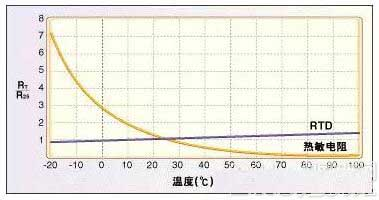In general, thermistors are a low-cost method of temperature measurement and are simple to use. Below we introduce the resistance temperature detector and the thermocouple temperature sensor.

Resistance temperature detector
A resistance temperature detector (RTD) is actually a special wire whose resistance varies with temperature. Usually RTD materials include copper, platinum, nickel and nickel/iron alloys. The RTD element can be a wire or a thin film coated on a ceramic substrate by electroplating or sputtering.
The resistance value of the RTD is a nominal value of 0 °C. 0°C 100Ω Platinum RTD resistance is typically 100.39Ω at 1°C and 119.4Ω at 50°C. Figure 4 is a comparison of the RTD resistance/temperature curve versus the thermistor resistance/temperature curve. The error of the RTD is smaller than that of the thermistor. For platinum, the error is generally 0.01%, and the nickel is generally 0.5%. In addition to the small error and resistance, the interface circuit of the RTD and the thermistor is basically the same.

Thermocouple
Thermocouples are made up of two different metals that generate a small voltage when heated. The magnitude of the voltage depends on the two metallic materials that make up the thermocouple. Iron-constantan (J-type), copper-constantan (T-type) and chrome-aluminum (K-type) thermocouples are the three most commonly used.
Thermocouples produce very small voltages, usually only a few millivolts. The voltage change of the K-type thermocouple temperature is only about 40μV when the temperature changes by 1 °C, so the measurement system should be able to measure the measurement accuracy of the voltage variation of 4μV to reach 0.1 °C.
Since the two different types of metals combine to create a potential difference, the connection of the thermocouple to the measurement system also produces a voltage. The connection point is usually placed on the insulating block to reduce this effect, so that the two nodes are at the same temperature, thereby reducing the error. Sometimes the temperature of the insulation block is also measured to compensate for the effects of temperature (Figure 5).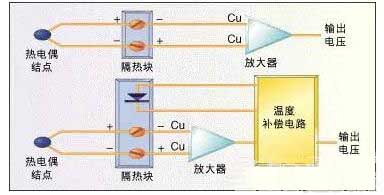The gain required to measure the thermocouple voltage is typically 100 to 300, and the noise drawn by the thermocouple is amplified by the same factor. A measurement amplifier is typically used to amplify the signal because it removes common mode noise from the thermocouple wires. Thermocouple signal conditioners, such as Analog Devices' AD594/595, are available on the market to simplify hardware interfaces.

Solid state thermal sensor
The simplest semiconductor temperature sensor is a PN junction, such as a PN junction between a diode or a transistor base-emitter. If a constant current flows through the forward biased silicon PN junction, the forward voltage drop is reduced by 1.8 mV for every 1 °C change in temperature. Many ICs use this characteristic of semiconductors to measure temperature, including Maxim's MAX1617, the country's LM335 and LM74. Semiconductor sensors come in a variety of interfaces, from voltage output to serial SPI/microwire interfaces.
There are many types of temperature sensors, and by properly selecting software and hardware, you can find the right sensor for your application.

﻿
﻿